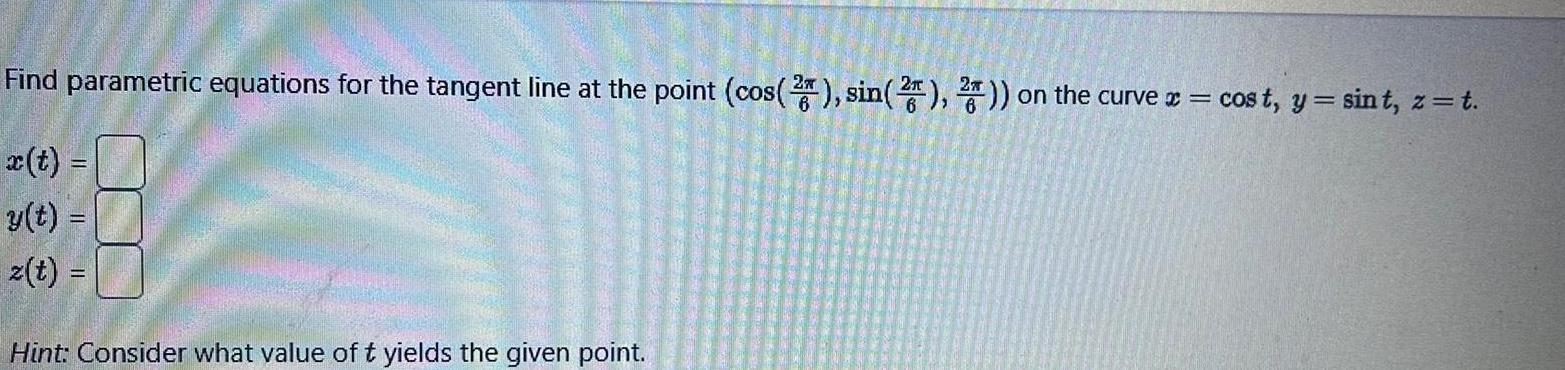Question:

# Find parametric equations for the tangent line at the point

Last updated: 11/21/2023Find parametric equations for the tangent line at the point cos 2 sin 2 2 on the curve x cost y sint z t y t z t Hint Consider what value of t yields the given point